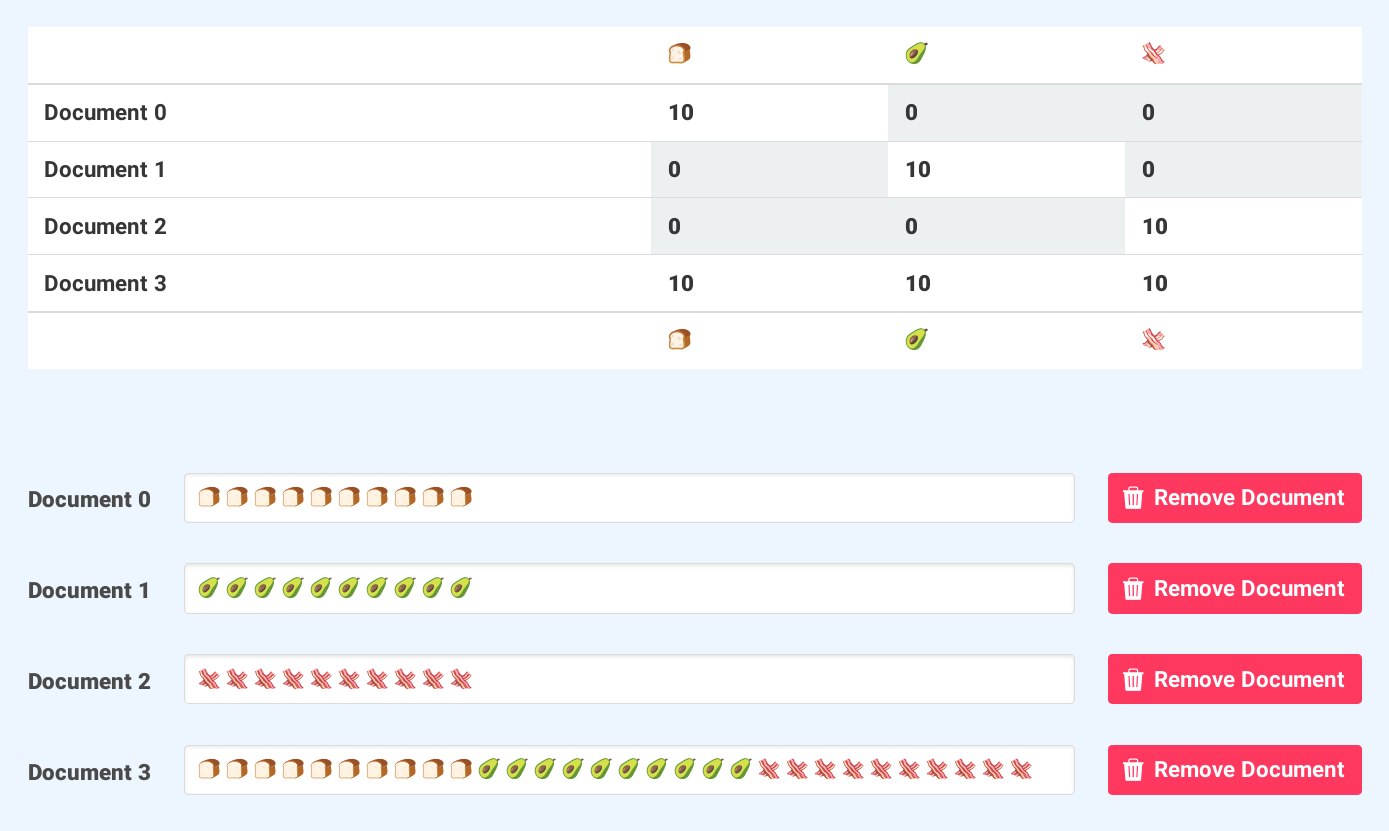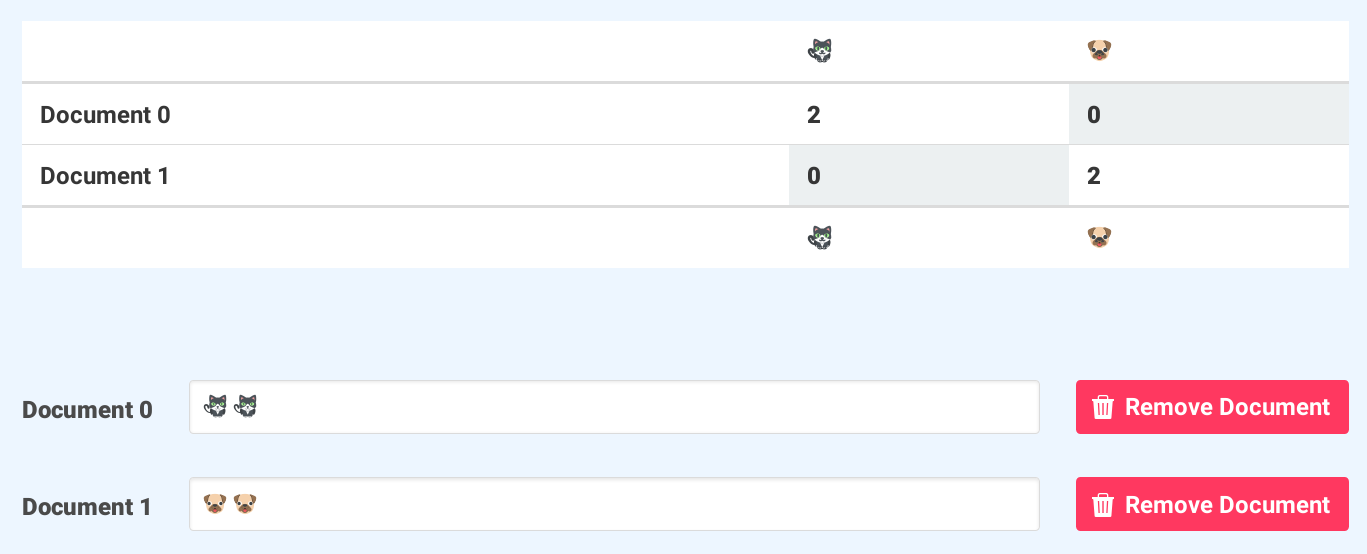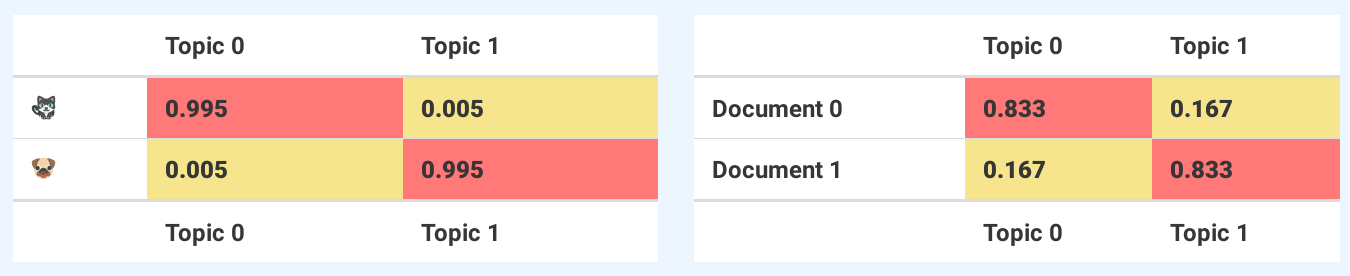# Your Guide to Latent Dirichlet AllocationNot too many stock photos for “Latent Dirichlet Allocation”.

Before we get started, I made a tool (here’s the source) that runs LDA right inside your browser (it’s pretty neat). Be sure to have that open as I go along, it will make things a lot clearer.

All of the emoji I use come from this page. Just select any one emoji and copy it into the input boxes. If you don’t see the emoji, try using Firefox version 50 or greater.

## What is LDA?

Latent Dirichlet Allocation (LDA) is a “generative probabilistic model” of a collection of composites made up of parts. Its uses include Natural Language Processing (NLP) and topic modelling, among others.

In terms of topic modelling, the composites are documents and the parts are words and/or phrases (phrases n words in length are referred to as n-grams).

But you could apply LDA to DNA and nucleotides, pizzas and toppings, molecules and atoms, employees and skills, or keyboards and crumbs.

The probabilistic topic model estimated by LDA consists of two tables (matrices). The first table describes the probability or chance of selecting a particular part when sampling a particular topic (category).

The second table describes the chance of selecting a particular topic when sampling a particular document or composite.I suddenly have a taste for bacon avocado toast.

Take the image up above for example. We have four emoji sequences (the ‘composites’) and three types of emoji (the ‘parts’).

The first three documents have ten of only one type of emoji, while the last document has ten of each type.

After we run our emoji composites through LDA, we end up with the probabilistic topic model you see above.

The left table has ‘emoji-versus-topics’, and the right table shows ‘documents-versus-topics’. Each column in the left table and each row in the right table sums to one (allowing for some truncation and precision loss).

So if I were to sample (draw an emoji out of a bag) Topic 0, I’d almost certainly get the avocado emoji. If I sampled Document 3, there’s an equal (or ‘uniform’) probability I’d get either Topic 0, 1, or 2.

The LDA algorithm assumes your composites were generated like so:

1. Pick your unique set of parts.
2. Pick how many composites you want.
3. Pick how many parts you want per composite (sample from a Poisson distribution).
4. Pick how many topics (categories) you want.
5. Pick a number between not-zero and positive infinity and call it alpha.
6. Pick a number between not-zero and positive infinity and call it beta.
7. Build the ‘parts-versus-topics’ table. For each column, draw a sample (spin the wheel) from a Dirichlet distribution (which is a distribution of distributions) using beta as the input. Each sample will fill out each column in the table, sum to one, and give the probability of each part per topic (column).
8. Build the ‘composites-versus-topics’ table. For each row, draw a sample from a Dirichlet distribution using alpha as the input. Each sample will fill out each row in the table, sum to one, and give the probability of each topic (column) per composite.
9. Build the actual composites. For each composite, 1) look up its row in the ‘composites-versus-topics’ table, 2) sample a topic based on the probabilities in the row, 3) go to the ‘parts-versus-topics’ table, 4) look up the topic sampled, 5) sample a part based on the probabilities in the column, 6) repeat from step 2 until you’ve reached how many parts this composite was set to have.

Now we know this algorithm (or generative procedure/process) is not how documents (such as articles) are written, but this — for better or worse — is the simplified model LDA assumes.

## What is the Dirichlet distribution?

A bit about the Dirichlet distribution before we move on.

What you see in the animation above are iterations of taking 1000 samples from a Dirichlet distribution using an increasing alpha value.

The Dirichlet distribution takes a number (called alpha in most places) for each topic (or category). In the GIF (and for our purposes), every topic is given the same alpha value you see displayed. Each dot represents some distribution or mixture of the three topics like (1.0, 0.0, 0.0) or (0.4, 0.3, 0.3). Remember that each sample has to add up to one.

At low alpha values (less than one), most of the topic distribution samples are in the corners (near the topics). For really low alpha values, it’s likely you’ll end up sampling (1.0, 0.0, 0.0), (0.0, 1.0, 0.0), or (0.0, 0.0, 1.0). This would mean that a document would only ever have one topic, if we were building a three topic probabilistic topic model from scratch.

At alpha equal to one, any space on the surface of the triangle (or if you prefer, ‘2-simplex’) is fair game (in other words, uniformly distributed). You could equally likely end up with a sample favoring only one topic, a sample that gives an even mixture of all the topics, or something in between.

For alpha values greater than one, the samples start to congregate in the center of the triangle. This means that as alpha gets bigger, your samples will more likely be uniform — that is, represent an even mixture of all the topics.

The GIF demonstrates the sampling of topic mixtures for the documents, but the Dirichlet distribution is also assumed the source (the Bayesian prior) for the mixture of parts per topic.

Three topics were used, as that works well when plotting in three dimensions. But typically, it is better to use more than three topics, depending on the number of documents you have.

If you look at the LDA tool mentioned earlier, you’ll see an alpha and beta slider.

These two hyperparameters are required by LDA. The alpha controls the mixture of topics for any given document. Turn it down, and the documents will likely have less of a mixture of topics. Turn it up, and the documents will likely have more of a mixture of topics.

The beta hyperparameter controls the distribution of words per topic. Turn it down, and the topics will likely have less words. Turn it up, and the topics will likely have more words.

Ideally, we want our composites to be made up of only a few topics and our parts to belong to only some of the topics. With this in mind, alpha and beta are typically set below one.

## Why use LDA?

If you view the number of topics as a number of clusters and the probabilities as the proportion of cluster membership, then using LDA is a way of soft-clustering your composites and parts.

Contrast this with say, k-means, where each entity can only belong to one cluster (hard-clustering). LDA allows for ‘fuzzy’ memberships. This provides a more nuanced way of recommending similar items, finding duplicates, or discovering user profiles/personas.

You could analyze every GitHub repository’s topics/tags and infer themes like native desktop client, back-end web service, single-paged app, or flappy bird clone.

If you choose the number of topics to be less than the documents, using LDA is a way of reducing the dimensionality (the number of rows and columns) of the original composite versus part data set.

With the documents now mapped to a lower dimensional latent/hidden topic/category space, you can now apply other machine learning algorithms which will benefit from the smaller number of dimensions. For example, you could run your documents through LDA, and then hard-cluster them using DBSCAN.

Of course, the main reason you’d use LDA is to uncover the themes lurking in your data. By using LDA on pizza orders, you might infer pizza topping themes like ‘spicy’, ‘salty’, ‘savory’, and ‘sweet’.

You could analyze every GitHub repository’s topics/tags, and infer themes like ‘native desktop client’, ‘back-end web service’, ‘single paged app’, or ‘flappy bird clone’.

## How does LDA work?

There are a few ways of implementing LDA. Still, like most — if not all — machine learning algorithms, it comes down to estimating one or more parameters.

For LDA, those parameters are phi and theta (although sometimes they’re called something else). Phi is the ‘parts-versus-topics’ matrix, and theta is the ‘composites-versus-topics’ matrix.Are you more a cat or dog person?

To learn how it works, let’s walk through a concrete example.

The documents and emojis are shown in the image above.

Our hyperparameters are:

• alpha = 0.5
• beta = 0.01
• ‘topics’ = 2
• ‘iterations’ = 1.

The following manual run-through is based on the academic paper ‘Probabilistic Topic Models’ by Steyvers & Griffiths.

Instead of estimating phi and theta directly, we will estimate the topic assignments for each of the four emoji using the Gibbs sampling algorithm. Once we have the estimated topic assignments, we can then estimate phi and theta.

To start, we need to randomly assign a topic to each emoji. Using a fair coin (sampling from a uniform distribution), we randomly assign to the first cat emoji ‘Topic 0’, the second cat ‘Topic 1’, the first dog emoji ‘Topic 1’, and the second dog ‘Topic 0’.

`|         | Cat 0 | Cat 1 | Dog 0 | Dog 1 ||---------|-------|-------|-------|-------|| Topic 0 | *     |       |       | *     || Topic 1 |       | *     | *     |       |`

This is our current topic assignment per each emoji.

`|     | Topic 0 | Topic 1 ||-----|---------|---------|| Cat | 1       | 1       || Dog | 1       | 1       |`

This is our current emoji versus topic counts.

`|            | Topic 0 | Topic 1 ||------------|---------|---------|| Document 0 | 1       | 1       || Document 1 | 1       | 1       |`

This our current document versus topic counts.

Now we need to update the topic assignment for the first cat. We subtract one from the emoji versus topic counts for Cat 0, subtract one from the document versus topic counts for Cat 0, calculate the probability of Topic 0 and 1 for Cat 0, flip a biased coin (sample from a categorical distribution), and then update the assignment and counts.

`t0 =(  (cat emoji with Topic 0 + beta)  /  (emoji with Topic 0 + unique emoji * beta))*(  (emoji in Document 0 with Topic 0 + alpha)  /  (emoji in Document 0 with a topic + number of topics * alpha)) =(  (0 + 0.01)  /  (1 + 2 * 0.01))*(  (0 + 0.5)  /  (1 + 2 * 0.5)) = 0.0024509803921568627t1 = ((1 + 0.01) / (2 + 2 * 0.01)) * ((1 + 0.5) / (1 + 2 * 0.5))   = 0.375p(Cat 0 = Topic 0 | *) = t0 / (t0 + t1) = 0.006493506493506494p(Cat 0 = Topic 1 | *) = t1 / (t0 + t1) = 0.9935064935064936`

After flipping the biased coin, we surprisingly get the same Topic 0 for Cat 0 so our tables before updating Cat 0 remain the same.

Next we do for Cat 1 what we did for Cat 0. After the flipping the biased coin, we get Topic 0 so now our tables look like so.

`|         | Cat 0 | Cat 1 | Dog 0 | Dog 1 ||---------|-------|-------|-------|-------|| Topic 0 | *     | *     |       | *     || Topic 1 |       |       | *     |       |`

This is our current topic assignment per each emoji.

`|     | Topic 0 | Topic 1 ||-----|---------|---------|| Cat | 2       | 0       || Dog | 1       | 1       |`

This is our current emoji versus topic counts.

`|            | Topic 0 | Topic 1 ||------------|---------|---------|| Document 0 | 2       | 0       || Document 1 | 1       | 1       |`

This our current document versus topic counts.

What we did for the two cat emoji we now do for the dog emoji. After flipping the biased coins, we end up assigning Topic 1 to Dog 0 and Topic 1 to Dog 1.

`|         | Cat 0 | Cat 1 | Dog 0 | Dog 1 ||---------|-------|-------|-------|-------|| Topic 0 | *     | *     |       |       || Topic 1 |       |       | *     | *     |`

This is our current topic assignment per each emoji.

`|     | Topic 0 | Topic 1 ||-----|---------|---------|| Cat | 2       | 0       || Dog | 0       | 2       |`

This is our current emoji versus topic counts.

`|            | Topic 0 | Topic 1 ||------------|---------|---------|| Document 0 | 2       | 0       || Document 1 | 0       | 2       |`

This our current document versus topic counts.

Since we’ve completed one iteration, we are now done with the Gibbs sampling algorithm and we have our topic assignment estimates.

To estimate phi, we use the following equation for each row-column cell in the ‘emoji-versus-topic’ count matrix.

`Phi row column =  (emoji row with topic column + beta)  /  (all emoji with topic column + unique emoji * beta)`

And for estimating theta, we use the following equation for each row-column cell in the document versus topic count matrix.

`Theta row column =  (emoji in document row with topic column + alpha)  /  (emoji in document row + number of topics * alpha)`Stylish styling brought to you by Bulma.

At long last we are done. The image above contains the estimated phi and theta parameters of our probabilistic topic model.

The matrix on the left shows us that the cat emoji is much, much more likely to represent Topic 0 than Topic 1, and that the dog emoji is much more likely to represent Topic 1 than Topic 0.

The matrix on the right shows us that Document 0 is more likely to be about Topic 0 than Topic 1, and that Document 1 is more likely to be about Topic 1.

This is how LDA can be used to classify documents (composites) and words/n-grams (parts) into topics.

If you enjoyed this article, you might also like Boost your skills, how to easily write K-Means, k-Nearest Neighbors from Scratch, and Let’s make a Linear Regression Calculator with PureScript.

How have you used LDA? Do you prefer Gensim or MALLET? How cool would it be if Elasticsearch did topic modeling out of the box? Let me know in the responses below. Thanks!

--

--